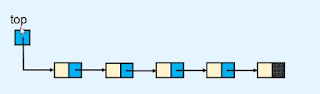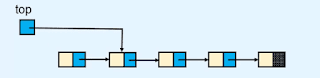The stack is an abstract type of data structure. it contains elements in linear order and we allow to insert and delete the data only at one end.

## Example of the stack in Data structures

as you see in the above image here is the stack of the plates. and in this stack, we can only insert a new plate from the top and can remove the plate from the top. so we only allow insert and remove the plate from the stack at the top end.

and it follows the LIFO property means last in first out. the element which is entered at the last can be deleted first. and we don't allow to perform operations in the middle area.

#### Push operation

to insert a new value or element in the stack is called a push operation.

#### Pop operation

to delete a value or element is called a pop operation.

#### Overflow state

when the stack becomes full and we don't allow to insert a new element in it. this condition or state is called the overflow state of the stack.

#### Underflow state

If we want to delete an element or value from an empty stack. this condition is called an underflow state of the stack.

#### Top-end

the end position from where we want to insert and delete the elements in the stack is called the top end of the list.

Example
if we implement a stack using the array and we want to insert and delete the element from the last position of the array then the last position is called the top end of the stack.

## Implementation of the stack

we can implement a stack using the array or linked list. first, let's see how we can implement a stack using an array.

### Implementation of stack using an array.

but before we implement a stack using an array we need to find the top position of the stack from where we can easily perform insertion and deletion operations in the stack.

if we take the first position as a top position of the stack then to insert a new value we need to push one step forward for every element. also to delete an element from the stack we need to push one step backward every element.

and if we take the last position of the array as the top position of the stack then we can easily insert and delete an element.

In the above example, we take an empty array. first, we insert or push the values 20, 30, 15, and 40 then we pop the last entered values 40 and 15. and after that, we push 56 into the stack, and then we pop 56 from the stack.

#### Program to Implementation of stack using an array in the python programming language.

```class EmptyStackError(Exception):
pass
class Stack:

def __init__(self):
self.items = []

def is_empty(self):
return self.items == []

def size(self):
return len(self.items)

def push(self, item):
self.items.append(item)

def pop(self):
if self.is_empty():
raise EmptyStackError("Stack is empty")
return self.items.pop()

def peek(self):
if self.is_empty():
raise EmptyStackError("Stack is empty")
return self.items[-1]

def display(self):
print(self.items)

if __name__ == '__main__':
st = Stack()

while True:
print("1. Push")
print("2. Pop")
print("3. Peek")
print("4. Size")
print("5. Display")
print("6. Quit")

choice = int(input("Enter your choice : "))

if choice == 1:
x = int(input("Enter the element to be pushed : "))
st.push(x)
elif choice == 2:
x = st.pop()
print("Popped element is : ", x)
elif choice == 3:
print("Element at the top is : ", st.peek())
elif choice == 4:
print("Size of stack ", st.size())
elif choice == 5:
st.display()
elif choice == 6:
break        else:
print("Wrong choice ")
print()

```

### Implementation of stack using a linked list.first, we need to find which end we need to choose to perform operations on the linked list so we can easily insert and deleter a new node or value in the stack.

if we choose the last position of the linked list as the top position of the stack then we need to traverse the whole list to insert and delete the node or value.

and if we choose the first position of the linked list as the top position of the stack then we don't need to traverse the whole list to insert and delete the node or value. so we choose the first position of the linked list as the top position to implement the stack.

#### Push operation

to perform a push operation in a stack we need to insert a new node at the beginning position of the linked list. and we already know how to insert a new node at the beginning of the linked list.

#### Pop operation

to perform the pop operation in a stack we need to delete the first node of the linked list. and we already know how to delete the first node of the linked list.if you don't know how to delete the first node of the linked list then check out the deletion in the linked list tutorial.

#### Program to implement a stack using a linked list in the python programming language.

```class EmptyStackError(Exception):
pass
class Node:

def __init__(self,value):
self.info = value
class Stack:

def __init__(self):
self.top = None
def is_empty(self):
return self.top == None
def size(self):

if self.is_empty():
return 0
count = 0        p = self.top
while p is not None:
count +=1            p = p.link
return count

def push(self,data):
temp = Node(data)
self.top = temp

def pop(self):
if self.is_empty():
raise EmptyStackError("Stack is empty")
return popped

def peek(self):
if self.is_empty():
raise EmptyStackError("Stack is empty")
return self.top.info

def display(self):
if self.is_empty():
print("Stack is empty")
return
print("Stack is :   ")
p = self.top
while p is not None:
print(p.info, " ")

if __name__ == '__main__':
st = Stack()

while True:
print("1. Push")
print("2. Pop")
print("3. Peek")
print("4. Size")
print("5. Display")
print("6. Quit")

choice = int(input("Enter your choice : "))

if choice == 1:
x = int(input("Enter the element to be pushed : "))
st.push(x)
elif choice == 2:
x = st.pop()
print("Popped element is : ", x)
elif choice == 3:
print("Element at the top is : ", st.peek())
elif choice == 4:
print("Size of stack ", st.size())
elif choice == 5:
st.display()
elif choice == 6:
break        else:
print("Wrong choice")
print()```